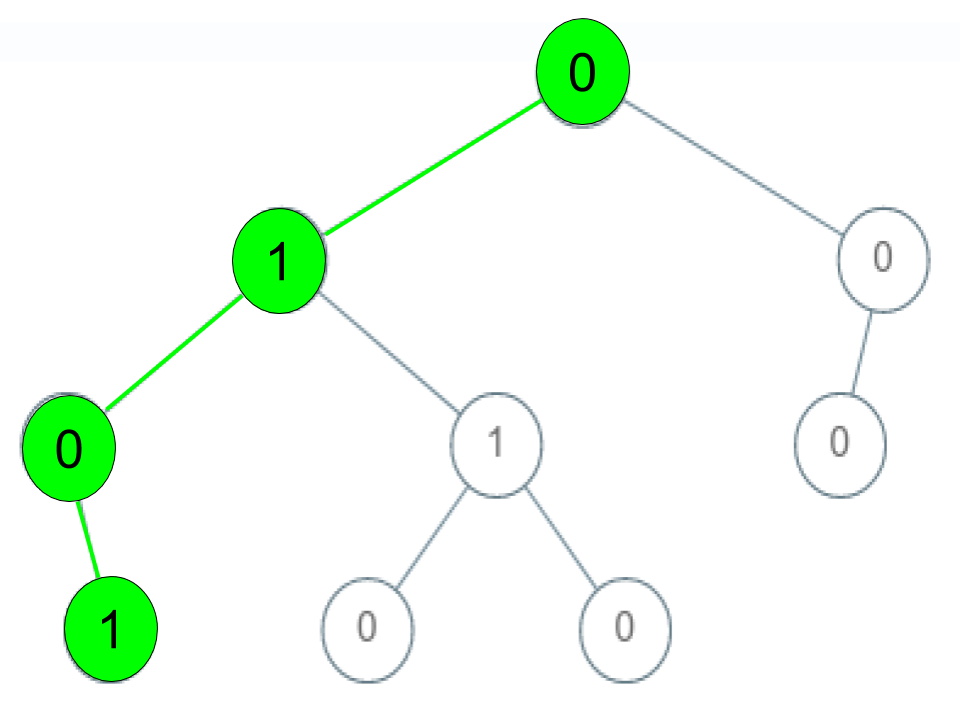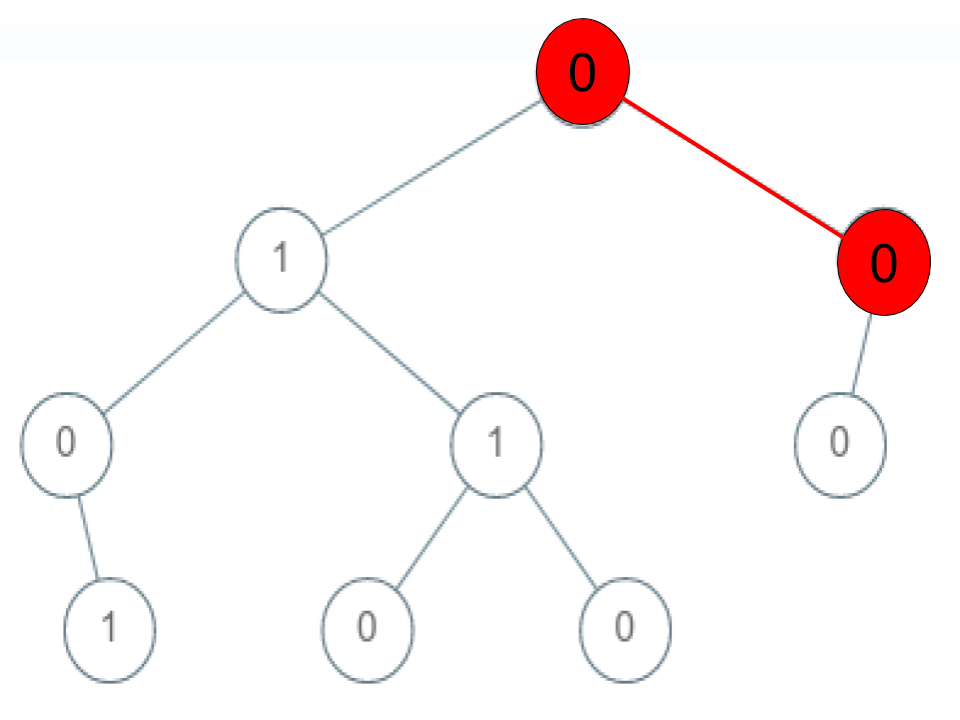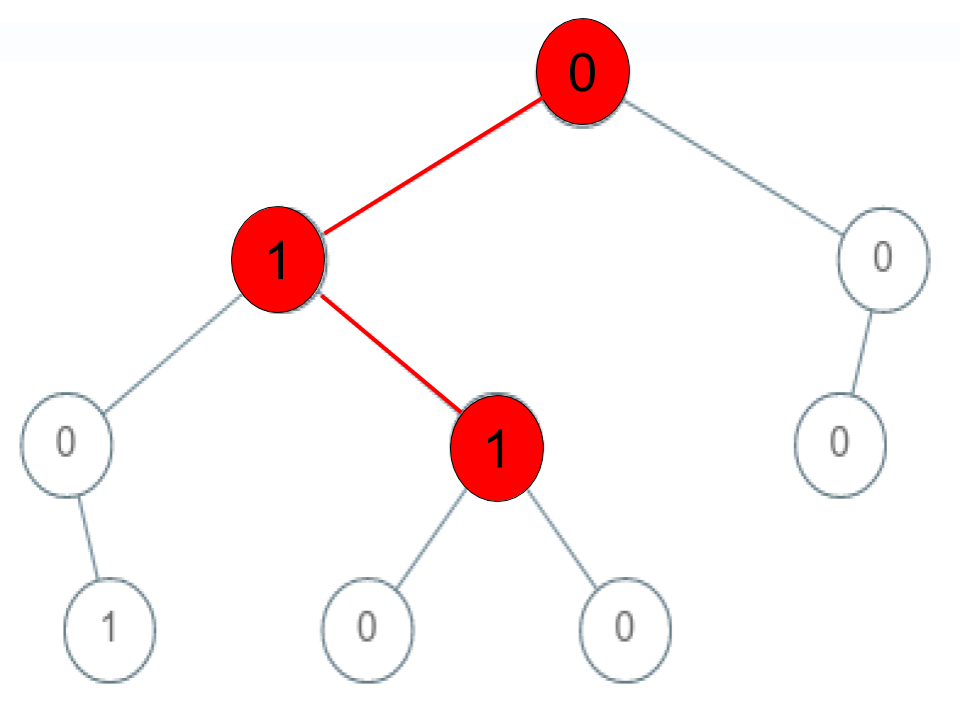Tags: "leetcode", "binary-tree", "dfs", access_time 2-min read

# Check if a String Is a Valid Sequence From Root to Leaves Path in a Binary Tree

#### Created: April 30, 2020 by [lek-tin]

##### Last updated: April 30, 2020

Given a binary tree where each path going from the root to any leaf form a valid sequence, check if a given string is a valid sequence in such binary tree.

We get the given string from the concatenation of an array of integers arr and the concatenation of all values of the nodes along a path results in a sequence in the given binary tree.

### Example 1:``````Input: root = [0,1,0,0,1,0,null,null,1,0,0], arr = [0,1,0,1]
Output: true
Explanation:
The path 0 -> 1 -> 0 -> 1 is a valid sequence (green color in the figure).
Other valid sequences are:
0 -> 1 -> 1 -> 0
0 -> 0 -> 0
``````

### Example 2:``````Input: root = [0,1,0,0,1,0,null,null,1,0,0], arr = [0,0,1]
Output: false
Explanation: The path 0 -> 0 -> 1 does not exist, therefore it is not even a sequence.
``````

### Example 3:``````Input: root = [0,1,0,0,1,0,null,null,1,0,0], arr = [0,1,1]
Output: false
Explanation: The path 0 -> 1 -> 1 is a sequence, but it is not a valid sequence.
``````

#### Constraints:

1. `1 <= arr.length <= 5000`
2. `0 <= arr[i] <= 9`
3. Each node’s value is between `[0 - 9]`.

### Solution

Java

``````/**
* Definition for a binary tree node.
* public class TreeNode {
*     int val;
*     TreeNode left;
*     TreeNode right;
*     TreeNode() {}
*     TreeNode(int val) { this.val = val; }
*     TreeNode(int val, TreeNode left, TreeNode right) {
*         this.val = val;
*         this.left = left;
*         this.right = right;
*     }
* }
*/
class Solution {
private boolean isValid = false;
public boolean isValidSequence(TreeNode root, int[] arr) {
helper(root, arr, 0);
return isValid;
}

private void helper(TreeNode root, int[] arr, int curr) {
if (isValid) return;
if (root == null) return;
if (root != null && curr == arr.length) return;

if (root.val != arr[curr]) return;

if (root.left == null && root.right == null && curr == arr.length-1) {
isValid = true;
} else {
helper(root.left, arr, curr+1);
helper(root.right, arr, curr+1);
}
}
}
``````